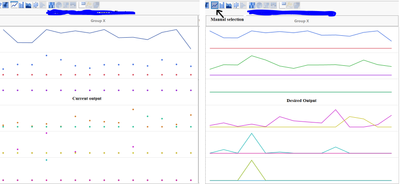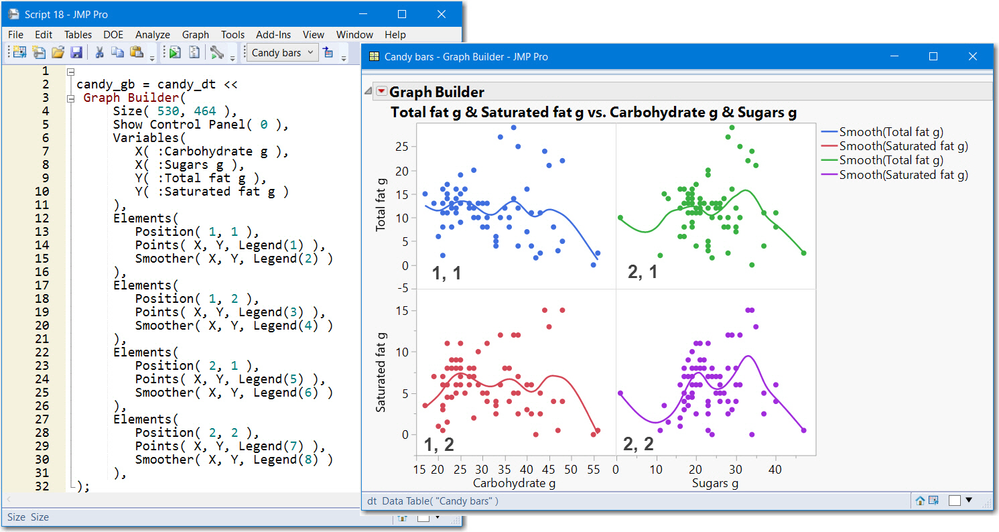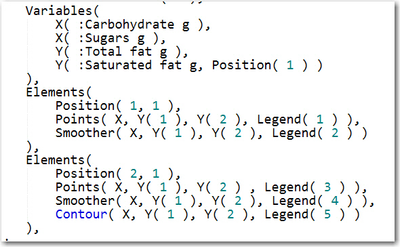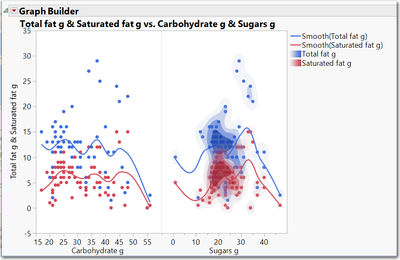Choose Language Hide Translation Bar
Highlighted

## How to make overlaid data sets show as a line instead of distinct points (via script)?

I am trying to get all of my data sets in a line (connection between each point) format. I am able to manually do this in the graph builder by clicking on the line indicator at the top of the graph indicator. However, I need to implement this function into the script. When I use the elements(line(x,y)) function, I only turn one data set (data set 1) into a line. The script I am using below shows what I input, and the pictures show what my output is vs. my desired output (all data shown as lines).

``````trend6 = Graph Builder(
Variables(
X( :Timestamp ),
Y( :data set 1 ),
Y( :data set 1b, Position(1)),
Y( :data set 2, Position(2) ),
Y( :data set 2b, Position(2)),
Y( :data set 3, Position(3)),
Y( :data set 3b, Position(3)),
Y( :data set 4, Position(4) ),
Y( :data set 4b, Position(4)),
Y( :data set 5, Position(5) ),
Y( :data set 5b, Position(5)),
Y( :data set 6, Position(6)),
Y( :data set 6b, Position(6)),
),elements(Line(X,Y)
));``````1 ACCEPTED SOLUTION

Accepted Solutions
Highlighted

## Re: How to make overlaid data sets show as a line instead of distinct points (via script)?

I'm not sure what elements does exactly; I tend to create graphs manually, get the script from the red triangle, and plug that into my main JSL program

If the number of Y variables is constant I would manually create the graph, click on the red triangle and copy the resultant script and reuse it.  If you have a varying number of Y variables you'll need to program the JSL logic dynamically so that it looks like what I created.  Just mimic the pattern using a loop.  If you need more help let us know.

4 REPLIES 4
Highlighted

## Re: How to make overlaid data sets show as a line instead of distinct points (via script)?

I created a small table with 1 x and 3 ys.  I used graph builder to plot x vs y1, y2 and y3, and manually selected lines for all x-y pairs.  This is the resulting JSL:

``````New Table( "Untitled 60", Add Rows( 4 ),
New Column( "x", Numeric, "Continuous", Format( "Best", 12 ), Set Values( [1, 2, 3, 4] ) ),
New Column( "y1", Numeric, "Continuous", Format( "Best", 12 ), Set Values( [5, 6, 7, 8] ) ),
New Column( "y2", Numeric, "Continuous", Format( "Best", 12 ), Set Values( [4, 5, 4, 5] ) ),
New Column( "y3", Numeric, "Continuous", Format( "Best", 12 ), Set Values( [3, 4, 3, 4] ) )
);

gb = Graph Builder( Show Control Panel( 0 ), Variables( X( :x ), Y( :y3 ), Y( :y2 ), Y( :y1 ) ), Elements( Position( 1, 1 ), Line( X, Y, Legend( 17 ) ) ), Elements( Position( 1, 2 ), Line( X, Y, Legend( 18 ) ) ), Elements( Position( 1, 3 ), Line( X, Y, Legend( 19 ) ) ));``````

Looks like you need to add some more Elements statements.  I'm using JMP 14.

Highlighted

## Re: How to make overlaid data sets show as a line instead of distinct points (via script)?

Thanks for the quick response. I'm unsure about how the elements statement works; what do the numbers in position(a,b) and legend(n) stand for? I'm not sure I need the legend; one is autogenerated in the graph builder.

Highlighted

## Re: How to make overlaid data sets show as a line instead of distinct points (via script)?

I'm not sure what elements does exactly; I tend to create graphs manually, get the script from the red triangle, and plug that into my main JSL program

If the number of Y variables is constant I would manually create the graph, click on the red triangle and copy the resultant script and reuse it.  If you have a varying number of Y variables you'll need to program the JSL logic dynamically so that it looks like what I created.  Just mimic the pattern using a loop.  If you need more help let us know.

Highlighted

## Re: How to make overlaid data sets show as a line instead of distinct points (via script)?

Maybe this will provide insight into elements. They specify the layout in matrix fashion. Note teh order of X's and Y's affect the display.Figure 6.25 from JSL Companion 2nd edition

This next example shows 2 X's and the x-axis is separate (not-merged), so there are 2 positions, 1,1 and 2,1 like a matrix.

Then you specify which GB components you want, smoothers, points, box plot, contours, whatever.

2 X's and 2 Y's with merged Y -axis Candy Bar DataTable 3.2 LeftTable 3.2 Right

Article Labels

There are no labels assigned to this post.